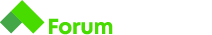# Distant Words

Здравейте на всички!

Имам проблем с outputa:

Примерът:

Input:

``````10
4
flower
window
curtain
book
``````

Output:

``````flower 69
window 78
curtain 76    //тук трябва да се натиска Enter, програмата да тръгне нататък.
//след натискането на Enter се принтира празната линия
book 33      //и най накрая се принтират останалите резултати.
64.0
``````

Output който трябваше:

### Output

``````flower 69
window 78
curtain 76
book 33
64.00
``````

Моля Помощ, ето го и кода ми:

Благодаря предварително!

====================================================

Angel and Bibkata have very weird taste - their last idea of fun is calculating the “distance” that a word is from a given number. The distance is calculated by summing the position in the alphabet of each letter in the word and than finding the absolute difference between that word and another predefined number. You are a programmer so you must ruin their fun by automating the process.

Examples:

• word ‘bob’, number = 22, distance = 3 (‘b’ + ‘o’ + ‘b’ = 2 + 15 + 2 = 19)
• word ‘bob’, number = 10, distance = 9

Write a program that calculates the distance for each string and also outputs the average distance.

## Input

• The input consists of several lines.
• T - the target number
• N - the number of words to follow
• on the next N lines - each word on a new line

## Output

• Output consists of N + 1 lines
• First N lines - word + its distance in format `word distance`
• Last line - the average distance, rounded to two digits after the decimal point

## Constraints

• Each word consists of only uppercase and lowercase english alphabet letters
• 1 <= N <= 20
• 0 <= T <= 1000

## Sample Tests

### Input

``````28
3
coffee
tea
pineapple
``````

### Output

``````coffee 12
tea 2
pineapple 66
26.67
``````

### Input

``````10
4
flower
window
curtain
book
``````

### Output

``````flower 69
window 78
curtain 76
book 33
64.00
``````

Решението ми е на C#, надявам се да помогне

``````int target = int.Parse(Console.ReadLine());
int sum = 0;
double avarage = 0;
for(int i = 0; i < count; i++)
{

for(int j = 0; j < word.Length; j++)
{
sum += word[j] - 96;
}
Console.WriteLine(word + " " + Math.Abs((target - sum)));
avarage += Math.Abs(target - sum);
sum = 0;
}
double avg = avarage / count;
Console.WriteLine(avg.ToString("N2"));
``````
1 Like

Здравей Мирослав и благодаря за споделеният код.

Корегирах кода ми, ама проблемът с outputa остана не решен, даже пробвах BufferedReader вместо Scanner, пак не става.

Аз реших да променя цялата концепция и понеже обичам да пиша методите, сега пробвам да го реша ето по този начин, ето го кода:

Поздрави!

Както при една друга задача, където ползвашe nextInt(), който не преминава на следващия ред, тук идва от next(), който има сходно поведение, само че вместо да чете “token”, в който да има само цифри, чете “token”, в който има само a-z символи. Съответно успява да открие всички такива token-и, като междувременно е прескочил всички символи за нов ред без последния, където зависва и се налага да терминираш с един допълнителен enter, както сам си открил.

1 Like

Здравей Едвард,
Аз го сменях на nextLine() и правеше същото, затова пробвах с BufferedReader(), чесно казано така и не успях да разбера какъв беше проблем, но самата задача я реших ето по този начин:

Иначе искам да реша и вторият вариант на решението който постнах по-нагоре (Distant Words_2), и който чуства сигурен e добре дошъл да контрибютне идеите/мислите си относно начините на имплементиране на метода:
printDistance(String[] array, int target)
че засега не успях да го имплементирам както трябва,
иначе
public static String[] getArrayOfStrings(int a)
е готов.

Хубав уикенд на всички!

Прави впечатление как в работещото решение ползваш именно nextLine().